# Decomposing the Variance in Reading Comprehension to Reveal the Unique and Common Effects of Language and Decoding

Behavior

## Your institution must subscribe to JoVE's Behavior section to access this content.

Fill out the form below to receive a free trial or learn more about access:

# Welcome!

Enter your email below to get your free 10 minute trial to JoVE!

We use/store this info to ensure you have proper access and that your account is secure. We may use this info to send you notifications about your account, your institutional access, and/or other related products. To learn more about our GDPR policies click here.

If you want more info regarding data storage, please contact gdpr@jove.com.

### Summary

Here we present a protocol for decomposing the variance in reading comprehension into the unique and common effects of language and decoding.

### Cite this Article

Copy Citation | Download Citations

Foorman, B. R., Petscher, Y. Decomposing the Variance in Reading Comprehension to Reveal the Unique and Common Effects of Language and Decoding. J. Vis. Exp. (140), e58557, doi:10.3791/58557 (2018).

### Abstract

The Simple View of Reading is a popular model of reading that claims that reading is the product of decoding and language, with each component uniquely predicting reading comprehension. Although researchers have argued whether the sum rather than the product of the components is the better predictor, no researchers have partitioned the variance explained to examine the extent to which the components share variance in predicting reading. To decompose the variance, we subtract the R2 for the language-only model from the full model to obtain the unique R2 for decoding. Second, we subtract the R2 for the decoding-only model from the full model to obtain the unique R2 for language. Third, to obtain the common variance explained by language and decoding, we subtract the sum of the two unique R2 from the R2 for the full model. The method is demonstrated in a regression approach with data from students in grades 1 (n = 372), 6 (n = 309), and 10 (n = 122) using an observed measure of language (receptive vocabulary), decoding (timed word reading), and reading comprehension (standardized test). Results reveal a relatively large amount of variance in reading comprehension explained in grade 1 by the common variance in decoding and language. By grade 10, however, it is the unique effect of language and the common effect of language and decoding that explained the majority of variance in reading comprehension. Results are discussed in the context of an expanded version of the Simple View of Reading that considers unique and shared effects of language and decoding in predicting reading comprehension.

### Introduction

The Simple View of Reading1 (SVR) continues as a popular model of reading because of its simplicity-reading (R) is the product of decoding (D) and language (L)-and because SVR tends to explain, on average, approximately 60% of explained variance in reading comprehension2. SVR predicts that correlations between D and R will decline over time and that correlations between L and R will increase over time. Studies generally support this prediction3,4,5. There are disagreements, however, about the functional form of SVR, with additive models (D + L = R) explaining significantly more variance in reading comprehension than product models (D × L = R)6,7,8, and a combination of sum and product [R = D + L + (D × L) explaining the largest amount of variance in reading comprehension3,9.

Recently the SVR model has expanded beyond regressions based on observed variables to latent variable modeling using confirmatory factory analysis and structural equation modeling. D is typically measured with untimed or timed reading of real words and/or nonwords and R is usually measured by a standardized reading test that includes literacy and informational passages followed by multiple-choice questions. L is typically measured by tests of expressive and receptive vocabulary and, especially in the primary grades, by measures of expressive and receptive syntax and listening comprehension. Most longitudinal studies report that L is unidimensional10,11,12,13. However, another longitudinal study14 reports a two-factor structure for L in the primary grades and a unidimensional structure in grades 4 and 8. Recent cross-sectional studies report that a bifactor model best fits the data and predicts R15,16,17,18. For example, Foorman et al.16 compared unidimensional, three-factor, four-factor, and bifactor models of SVR in data from students in grades 4-10 and found that a bifactor model fit best and explained 72% to 99% of the variance in R. A general L factor explained variance in all seven grades and vocabulary and syntax uniquely explained variance only in one grade each. Although the D factor was moderately correlated with L and R in all grades (0.40-0.60 and 0.47-0.74, respectively), it was not uniquely correlated with R in the presence of the general L factor.

Even though latent variable modeling has expanded SVR by shedding light on the dimensionality of L and the unique role that L plays in predicting R beyond the primary grades, no studies of SVR except one by Foorman et al.19 have partitioned the variance in reading comprehension into what is due uniquely to D and L and what is shared in common. This is a big omission in the literature. Conceptually it makes sense that D and L would share variance in predicting written language because word recognition entails the linguistic skills of phonology, semantics, and discourse at the sentence and text levels20. Similarly, linguistic comprehension must be connected to orthographic representations of phonemes, morphemes, words, sentences, and discourse if text is to be understood21. Multiplying D by L does not yield the knowledge shared by these components. Only decomposition of the variance into what is unique and what is shared by D and L in predicting R will reveal the integrated knowledge crucial to the success of educational interventions.

The one study by Foorman et al.19 that decomposed the variance of reading comprehension into what is unique and what is shared in common by D and L employed a latent variable modeling approach. The following protocol demonstrates the technique with data from students in grades 1, 7, and 10 based on single observed variables for D (timed decoding), L (receptive vocabulary), and R (standardized reading comprehension test) to make the decomposition process easy to understand. The data represent a subset of the data from Foorman et al.19.

Subscription Required. Please recommend JoVE to your librarian.

### Protocol

Note: The steps below describe decomposing total variance in a dependent variable (Y) into unique variance, common variance, and unexplained variance components based on two selected independent variables (calledand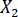for this example) using software with a graphical user interface and data management software (see Table of Materials).

1. Reading Data into Software with a Graphical User Interface

1. Click on File.
1. Hover the mouse over Open.
2. Click on Data.
2. Locate the relevant data file on the computer.
1. If the file type is not consistent with the software with a graphical user interface, click on Files of Type and select the appropriate file format.
3. Click on Open.

2. Estimate the Variance Explained in the Dependent Variable (Y)

1. Total Variance Explained based on Two Independent Variables — Total R2.
Note: An R2 value is known as the coefficient of determination and represents the proportion of variance for a dependent variable that is explained by a set of independent variables.
1. Click on Analyze and hover the mouse over Regression and select Linear.
2. Click on the dependent variable in the variable list. Then click on the arrow next to Dependent.
3. Click on the two independent variables (X1 and X2) in the variable list. Then click on the arrow next to Independent(s).
4. Click OK.
5. Click on the viewer window of the software.
1. Use the mouse to scroll to the section called Model Summary. Record the value under the column R Square and label this value Total R2.
2. Total Variance Explained based on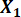1. Repeat steps 2.1.1 through 2.1.4 using onlyin the independent variable list.
2. Click on the viewer window of the software.
1. Use the mouse to scroll to the section called Model Summary. Record the value under the column R Square and label this valueR2.
3. Total Variance Explained based on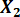1. Repeat steps 2.1.1 through 2.1.4 using onlyin the independent variable list.
2. Click on the viewer window of the software.
1. Use the mouse to scroll to the section called Model Summary. Record the value under the column R Square and label this valueR2.

3. Computing the Unique, Common, and Unexplained Variance Components

1. Open the data management software.
2. Enter the labels Total R2,R2, andR2 in cells A1, B1, and C1, respectively.
3. Enter the Total R2 value from step 2.1.5.1 in cell A2.
4. Enter theR2 value from step 2.2.2.1 in cell B2.
5. Enter theR2 value from step 2.3.2.1 in cell C2.
6. Calculate the Unique Variance of Variable 1 (UR2) in the data management software.
1. In Cell D2 type: “=A2-C2” (i.e., Total R2 minusR2). In Cell D1 label this value UR2.
7. Calculate the Unique Variance of Variable 2 (UR2) in the data management software.
1. In Cell E2 type: “=A2-B2” (i.e., Total R2 minusR2). In Cell E1 label this value UR2.
8. Calculate the Common Variance between Variables 1 and 2 (C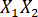R2) in the data management software.
1. In Cell F2 type: “=A2-D2-E2” (i.e., Total R2 minus UR2 minus UR2). In Cell F1 label this value CR2.
9. Calculate the Unexplained Variance (e) in the data management software.
1. In Cell G2 type: “=1-A2” (i.e., 1-Total R2). In Cell G1 label this value e.

4. Plot the UX1R2, UX2R2, CX1X2R2, and e values

Note: Values in cells D2, E2, F2, and G2 are plotted.

1. Click and drag the mouse over Cells D2, E2, F2, and G2 to highlight the data.
2. Click on Insert on the ribbon of the data management software.
3. Click on Charts | Pie Chart | 2-D Pie Chart.

Subscription Required. Please recommend JoVE to your librarian.

### Representative Results

The objective of this study was to investigate the contributions of unique and common variance of language (L) and decoding (D) to predicting reading comprehension (R) in grades 1, 7, and 10 in Florida, a state whose demographics are representative of the nation as a whole. There were two hypotheses regarding predictions of the variance explained in reading comprehension. First, after the primary grades, the unique contribution of D will significantly decrease, and the unique contribution of L will increase. Second, the unique contribution of L and the shared contributions of D and L will significantly account for the majority of variance beyond the primary grades.

Participants were 372 students in grade 1, 299 students in grade 7, and 122 students in grade 10 in general education classrooms from 18 schools in two large urban districts in Florida (one in northern Florida and the other in central Florida). The study followed guidelines for human subjects and parental consent was obtained. The ethnicity breakdown across grades for the study was: Approximately 30% Black; 30% Hispanic; 30% White; 5% Asian, 3% multicultural; 2% Other. The range of participation in the federal lunch program at the 18 participating schools was from 21.5% to 100%, with a median of 59%.

Single, observable measures for D, L, and R were selected for the regression analyses. The measure of decoding was time-limited (45 s) sight word decoding from the Test of Word Reading Efficiency-222. L was measured by a receptive vocabulary test, the Peabody Picture Vocabulary Test (PPVT-4)23, widely used in the participating schools. In this measure, students see four pictures and point to the one that depicts the word the examiner says. R was assessed with a nationally-normed reading comprehension test, the Gates-MacGinitie Reading Test-4 (GMAT-4)24. The GMAT-4 is administered in small groups of 10 students in grade 1. Students read parts of a passage and indicate the picture that corresponds to the passage. The GMAT-4 is group-administered in grades 7 and 10. Passages consist of both literary and informational text and questions are both literal and inferential and appear in a multiple-choice format. Students can look back at the passage. For all three measures, coefficients for reliability were above 0.90. A planned missing data design with three forms was used to reduce testing time. The D and L measures were administered in one session and the reading comprehension test in another session.

The regression analysis for grade 1 accounted for 60% of the total variance in reading comprehension. The individual variance models showed that the proportion of variance in reading comprehension due to D was 43% and that separately, the proportion of variance in reading comprehension due to L was 36%. These variance estimates are the squared correlation from separate statistical models of each predictor and outcome, which is why their sum from separate models (43 + 36 = 79) was greater than the total amount of variance explained (60%). When the total variance in grade 1 was decomposed into unique and common effects, D uniquely explained 24% of the variance in R and L uniquely explained 17% (see Figure 1). The common variance of D and L was 19%.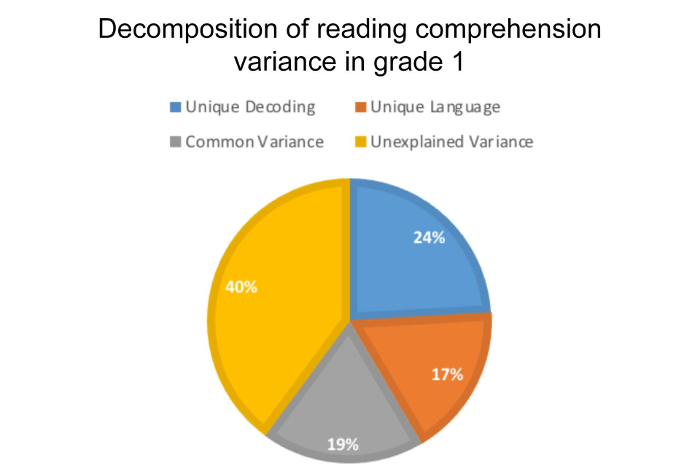Figure 1. Total percent of variance explained in grade 1 reading comprehension decomposed into unique and common effects of language and decoding and unexplained variance. Please click here to view a larger version of this figure.

In grade 7, the regression analysis accounted for 53% of the total variance in reading comprehension. The individual variance models showed that the proportion of variance in reading comprehension due to D was 25% and the proportion of variance in reading comprehension due to L was 46%. Figure 2 shows that D uniquely explained 7% of the variance in R and that L explained 28%. The common variance of D and L in explaining variance in R was 18%.Figure 2. Total percent of variance explained in grade 7 reading comprehension decomposed into unique and common effects of language and decoding and unexplained variance. Please click here to view a larger version of this figure.

In grade 10, the regression analysis accounted for 61% of the total variance in reading comprehension. The individual variance models showed that the proportion of variance in reading comprehension due to D was 19% and the proportion of variance in reading comprehension due to L was 54%. Figure 3 shows that D uniquely accounted for 6% of the variance, whereas L uniquely accounted for 42% of the variance. The common variance of D and L in explaining variance in R was 13%.Figure 3. Total percent of variance explained in grade 10 reading comprehension decomposed into unique and common effects of language and decoding and unexplained variance. Please click here to view a larger version of this figure.

Subscription Required. Please recommend JoVE to your librarian.

### Discussion

There are three critical steps in the protocol for decomposing the variance in R into unique and common variance due to L and D. First, subtract the R2 in the L-only model from the full model to obtain the unique R2 for D. Second, subtract the R2 for the D-only model from the full model to obtain the unique R2 for L. Third, to obtain the common variance explained by L and D, subtract the sum of the two unique R2 from the R2 for the full model.

Modifications to the protocol would be necessary if latent variables for D and L replaced the dummy codes for the observed measures of timed decoding and receptive vocabulary used here and if control variables such as socio-economic status (SES), gender, and race/ethnicity are added to the model. Alternatives to plotting the results in pie charts can also be considered, such as using Venn diagrams. Pie charts were used here so that percentages of unexplained variance as well as unique and common variances could be displayed.

There are limitations to the application of the method as shown in this study. To simplify the protocol, we selected one observable measure each for D, L, and R instead of using the latent variable modeling approach we usually take to control measurement error19. We eliminated control variables such as SES, gender, and race/ethnicity and used cross-sectional data with a planned missing data design rather than complete longitudinal data. We focused on decomposing variance at the individual student level rather than clustering students within classrooms and schools. Finally, the method shown in the protocol for decomposing variance into percentages of unique and common effects of L and D in predicting R yields descriptive results. There is no easy way to obtain a formal statistical test of the significance of the common variance.

This technique for decomposing the variance in R into the unique and common effects due to L and D has significant advantages over existing methods of looking solely at unique effects. Most importantly, the technique illustrates how individual difference characteristics covary and how one unique effect may pale in comparison to the effect shared with another characteristic. The analyses resulting from the current protocol showed that substantial amounts of variance in reading comprehension were due to the common effects of D and L (ranging from 19% in grade 1 to 13% in grade 10) that appeared to come at the expense of the unique contribution in D over the grades. In other words, the regression results showed a decline in the proportion of variance accounted for by D from 43% in grade 1 to 25% in grade 7 to 19% in grade 10. However, when the variance was decomposed, the unique contribution of D in grade 1 was only 24% and that declined in grades 7 and 10 to 7% and 6%, respectively. This finding has important educational implications because the emphasis on decoding in interventions in the elementary grades comes from the unique effect of D in regression results in spite of the weak effects of decoding interventions in the upper elementary and secondary grades in a meta-analysis25. The amount of common variance that D and L together explain in predicting reading comprehension, especially in the elementary grades, suggests that more instructional emphasis should be placed on the integration of linguistic knowledge at the word-level26,27.

Regression results for L showed a fairly constant picture of L contributing substantial proportions of variance to reading comprehension across the grades, 36% in grade 1 to 54% in grade 10. However, when the method of decomposing the variance was used, the unique contribution of L over the grades showed a dramatic increase from 17% in grade 1 to 28% in grade 7, to 42% in grade 10. The finding that L accounts for so much variance in R in the secondary grades is even more apparent in the SVR studies conducted from a latent variable modeling approach16,17,19 and suggests the value of instruction on the linguistic elements that make text cohesive26,28.

Subscription Required. Please recommend JoVE to your librarian.

### Disclosures

The authors declare that they have no competing financial interests.

### Acknowledgments

The research reported here was supported by the Institute of Education Sciences, U.S. Department of Education, through a subaward to Florida State University from Grant R305F100005 to the Educational Testing Service as part of the Reading for Understanding Initiative. The opinions expressed are those of the authors and do not represent views of the Institute, the U.S. Department of Education, the Educational Testing Service, or Florida State University.

### Materials

 Name Company Catalog Number Comments IBM SPSS Statistics Software IBM Microsoft Office Excel Microsoft

### Post a Question / Comment / Request

You must be signed in to post a comment. Please or create an account.